Related Articles

# Class 8 NCERT Solutions – Chapter 4 Practical Geometry – Exercise 4.4

• Last Updated : 15 Dec, 2020

### Question 1: Construct the following quadrilaterals.

DE = 4 cm
EA = 5 cm
AR = 4.5 cm
∠E = 60°
∠A = 90°

Solution:

Steps of construction:

Step 1: Draw a line segment DE of 4 cm.
Step 2: Construct an angle of 60° at E.
Step 3: From E draw an arc of 5 cm and intersect the arc with the angle line, mark the intersection point as A.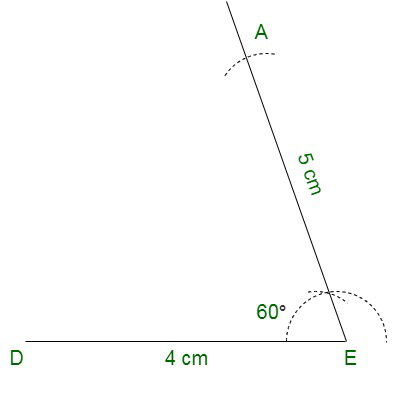Step 4: Draw a 90° angle at A.
Step 5: Draw an arc of 4.5 cm from A and cut the angle line at R.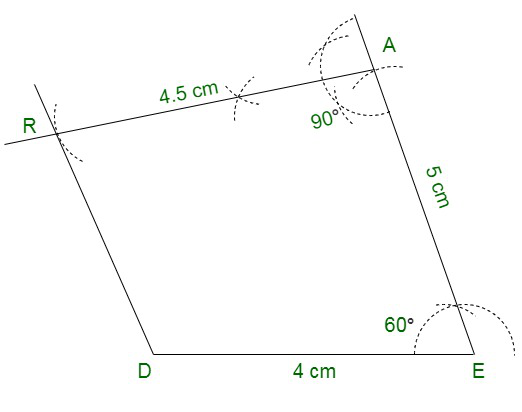Step 6: Join DR.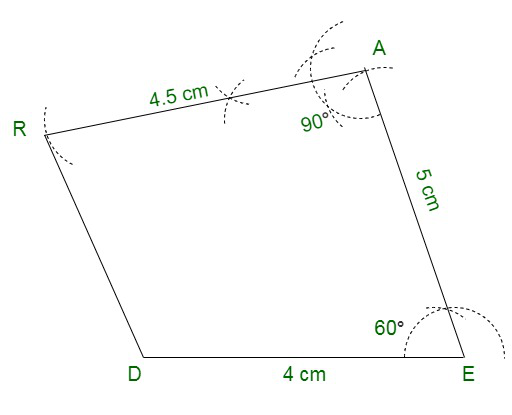Hence, we have the required quadrilateral DEAR.

TR = 3.5 cm
RU = 3 cm
UE = 4 cm
∠R = 75°
∠U = 120°

Solution:

Steps of construction:

Step 1: Draw a line TR of 3.5 cm.
Step 2: From R construct an angle of 75°.
Step 3: Draw an arc of 3 cm from R and cut the angle line at U.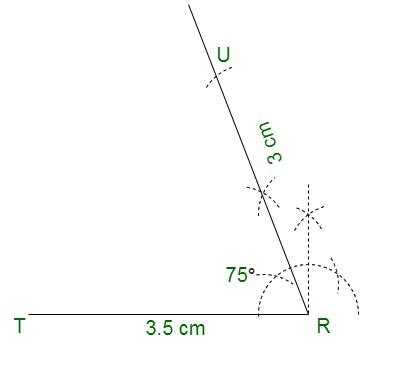Step 4: From U construct an angle of 120
Step 5: Draw an arc of 4 cm from U and cut the angle line at E.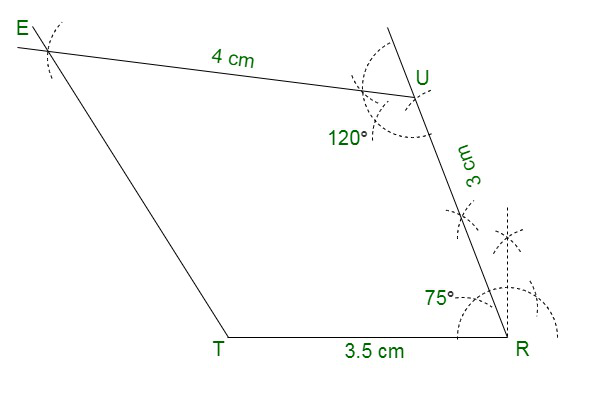Step 6: Join TE.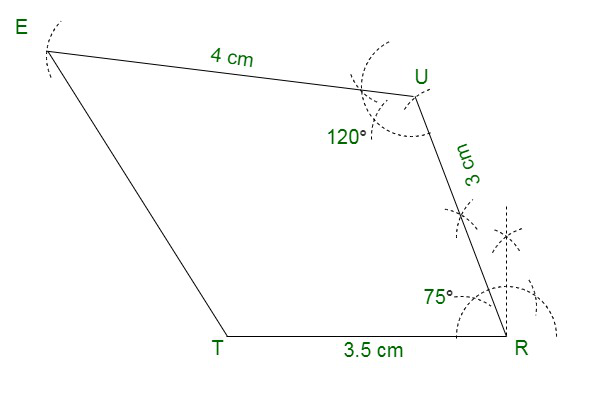Hence we have the required Quadrilateral TRUE.

Attention reader! All those who say programming isn’t for kids, just haven’t met the right mentors yet. Join the  Demo Class for First Step to Coding Coursespecifically designed for students of class 8 to 12.

The students will get to learn more about the world of programming in these free classes which will definitely help them in making a wise career choice in the future.

My Personal Notes arrow_drop_up